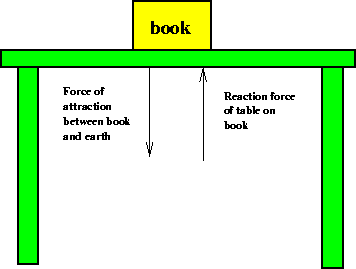Next: Weight and Mass Up: Newton's Laws Previous: Newton's 2nd Law

## Newton's 3rd Law

Newton's 2nd law deals with a single object on which a force is exerted. The 3rd and last law of motion discovered by Newton explains what happens to the object that is exerting the force. The 3rd law can be summarized by stating that:This tells us that the object exerting the force feels a reaction'' force with the same magnitude, but in the opposite direction from the force that it is exerting. Two examples of this law are as follows. Imagine pushing on a (solid) wall with a fairly large force, and assume you don't break through. According to Newton's third law, the wall is exerting an equal and opposite force back on you. How is this consistent with the 2nd law, which says a force causes an acceleration? The answer lies in the fact that as you push on the wall, and the wall pushes back on you, the friction between your shoes and the floor also exerts a force on you, which, if you are lucky, is just enough to keep you from moving backwards away from the wall. If you push against a wall while standing on ice, the frictional force is much less and you do in fact accelerate backwards away from the wall.

Imagine a slightly different scenario. Suppose you are taking a space walk near the space shuttle, and your safety line breaks. How would you get back to the shuttle? The thing to do would be to take one of the tools from your tool belt and throw it is hard as you can directly away from the shuttle. Then, with the help of Newton's second and third laws, you will accelerate back towards the shuttle. As you throw the tool, you push against it, causing it to accelerate. At the same time, by Newton's third law, the tool is pushing back against you in the opposite direction, which causes you to accelerate back towards the shuttle, as desired. This is the principle behind jet propulsion: a jet rocket expels fuel rapidly by pushing against it''. By the third law the fuel exerts an equal and opposite force against the rocket so the rocket accelerates in the opposite direction that the expelled fuel goes. Another example of the 3rd law is a book lying on a table. If the table were not there the book would fall under the force of gravity between the book and the earth. Why then doesn't the book move off the table, in accordance with the 2nd law? The table is exerting a force on the book that is just enough to cancel the gravitational force on the book. By Newton's third law, the book is also exerting an equal and opposite force on the table, and this is why the table feels the weight of the book. If the book were very heavy, its weight would cause the table to bend, or even break. This is illustrated in the following figure.Next: Weight and Mass Up: Newton's Laws Previous: Newton's 2nd Law
modtech@theory.uwinnipeg.ca
1999-09-29